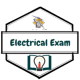# SSC JE 2009 Electrical question paper with Explained Solution

Ques 1. The value of current I flowing in the 1Ω resistor in the circuit shown in the figure below will be1. 10A
2. 6A
3. 5A
4. 0

When only voltage source is present then voltage across 1Ω resistor isV = 5V

I = V/R = 5/1 = 5A

Ques 2. In the figure shown below, if we connect a source of 2V, with internal resistance of 1Ω at AA’ with positive terminal at A, then the current through R is1. 2A
2. 1.66 A
3. 1 A
4. 0.625 A

Connect a source of 2V, with internal resistance of 1Ω at AA’Applying Nodal analysis at C and BSolving equation 1 & 2

VC = 1.125 Volt

VB = 1.25 Volt

Current through resistor R

IR = VB/2 = 1.25/2 = 0.625 A

Ques 3. The curve representing Ohm’s law is

1. Linear
2. Hyperbolic
3. Parabolic
4. Triangular

Ohm’s law of constant proportionality state that for a given conductor the ratio of voltage to current is constant i.e if the temperature, area, the length did not change the resulting current is directly proportional to the variation in the applied voltage. Hence the graph between voltage and current is linear.
R(constant) = V/I★Ohms law does not apply to all material it only applied to “linear” or “Ohmic Resistor”.

Ques 4. Specific resistance of a conductor depends upon

1.  Dimension of the conductor
2.  Composition of conductor material
3.  Resistance of the conductor
4.  Both (a) and (b)

For any given material at a certain given temperature, the resistance is given aswhere ρ is a constant depending on the nature of the material of the conductor and is known as its specific resistance or resistivity.

Specific Resistance depends only on temperature and material of the conductor but not on its dimensions of the conductor, on which resistance depends, and mechanical deformation such as stretching etc. As ρ depends only on the material of a conductor at a given temperature, hence it is a characteristic constant.

Ques 5. Superposition theorem is essentially based on the concept of

1. Reciprocity
2. Linearity
3. Duality
4. Non-linearity

According to superposition theorem

In any Linear and bilateral network consisting linear and bilateral and energy sources (e.g. generators), the current flowing at any point in the network is the algebraic sum of the currents those flow with each source were considered separately, with all the remaining source replaced at a time by their internal impedances.

The theorem is hence based on the linearity property and Ohm‘s law. The fundamental concept is that in linear impedance, for an increase in voltage across it, the current through it increases which is independent of the magnitude of the original current ﬂowing through it.

Ques 6. If a 500 KVA, 200 Hz transformer is operated at 50 Hz, its KVA rating will be

1. 2000 kVA
2. 125 kVA
3. 250 kVA
4. 1000 kVA

S ∝ induced EMF

S ∝ 4.44fNΦ

∴ S ∝ f

S1/S2 = f1/f2

S2 = S1f2/f1

= 500 x 50/200 =125 KVA

Ques 7. The angle between induced emf and terminal voltage on no-load for a single phase alternator is

1. 180°
2. 90°
3. 270°

The generated voltage of the alternator is

V = E – Ia(Ra + JXs)

Where E = Induced EMF

Ia = Armature current

Ra = Armature resistance

Xs = leakage reactance of the armature winding

For no-load armature current Ia = 0

∴ V = E  (δ = 0°)

So at no load, the angle between induced emf and terminal voltage is 0°

Ques 8. A salient pole synchronous generator connected to an infinite bus power will deliver maximum power at power angle of

1.  δ = 0°
2. δ = 90°
3. δ = 45°
4. δ = 30°

Power delivered by synchronous generatorFor Pmax  sinδ = 1

δ = 90°

Pmax = VE/X

Ques 9. Starting torque of synchronous motor is

1. Very low
2. Zero
3. Very high

The starting torque of the synchronous Motor is zero.Consider the rotor is at rest and the stator field is rotating at the synchronous speed at 100% slip. Suppose N pole of the rotating field in the stator is approaching at S pole of the rotor then the magnetic force will tend to turn the rotor in the direction opposite to the rotating field When the same N pole of the rotating field has just passed the rotor S pole, the magnet force will tend to pull the rotor in the same direction as the rotating field. These opposite interactions balance out, producing a zero net torque on the rotor.

Ques 10. The power factor at which the transformer operates

1. Is unity
2. is 0.8 lag
4. Depends upon the power factor of the load

The operating power factor in the case of a transformer depends upon the load supplied by the transformer. For example, if the transformer is supplying lamp load, the operating power factor is unity, whereas while in most industries, a large percentage of the load consists of an induction motor. These machines operate with lagging power factor hence the power factor of the transformer is lagging, Moreover, the magnitude of lagging power factor also depends on the nature of inductive load being supplied by the transformer Thus the transformer rating is always specified in terms of volt-ampere (VA) or Kilo-Volt-ampere KVA.

### 4 thoughts on “SSC JE 2009 Electrical question paper with Solution”

1.Thank you sir

1.Welcome Rupa Kumari

2.3.